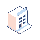• Fiyatlar

# How to compute the sum of order line field and place it in sale order?

I have a sale.order.line field commission_line that computes the commission amount of the product line.

`class SaleOrderlineInherited(models.Model):    _inherit = 'sale.order.line'    commission_line_percentage = fields.Float(related='product_id.product_tmpl_id.commission_percentage')    commission_line = fields.Float(string='Commission', compute='get_commission')    @api.depends('commission_line_percentage')        def get_commission(self):        for record in self:            record['commission_line'] = (record.price_subtotal * record.commission_line_percentage) / 100        return None`

The above code runs fine. Next I want to calculate all the sum of commission_line fields to get the total commission from the sale.order. I tried this code.

`class CustommSaleOrder(models.Model):    _inherit = 'sale.order'    commission = fields.Char(string='Total Commission', compute='calculate_total_commission')    def calculate_total_commission(self):        print 'came in'        summ = None        for record in self.env['sale.order.line']:            print 'commission'            summ += record.commission_line_percentage        self.env['sale.order'].commission = summ        return None`

This doesn't work. How to correct the code so as to get the sum of all commission_line as the total commission?##### 4 Yanıtlar

Hi,

Try like this,

`@api.depends('order_line.commission_line')def _commission_amount(self):      for order in self:              comm_total = 0.0               for line in order.order_line:                   comm_total += line.commission_line            order.update({'commission': comm_total })`

ThanksTry

@api.depends('order_line')
def _commission_amount(self):
for order in self:
comm_total = 0.0
for line in order.order_line:
comm_total += line.commission_lineNeither of the above worked for me. I created a float variable x_studio_nett_untaxed_total_1 with Related field order_line.x_studio_nett_total - another field I created. It is only using the first line of the order as the total. I have tried both ways above.

Related Field : order_line.x_studio_nett_total

De[endencies : order_line.x_studio_nett_total, x_studio_nett_untaxed_total_1

Computed :

@api.depends('order_line.x_studio_nett_total')

def _line_total(self) :

for order in self:

line_total = 0.0

for line in order.order_line:

line_total += line.x_studio_nett_total

order.update({'x_studio_nett_untaxed_total_1':line_total})Sorry for off topic! @Niyas, you are the best! I applied the formula on the total discount for SO with computed field. Sharing for reference...

float field on sale.order.line, x_price_discount_amount;

float field on sale.order, x_price_discount_amount_total, depends=order_line.x_price_discount_amount

`for record in self:  discount_total = 0.0  for line in record.order_line:      discount_total += line.x_price_discount_amount  record['x_price_discount_amount_total'] = discount_total`

It works! Thanks so much.